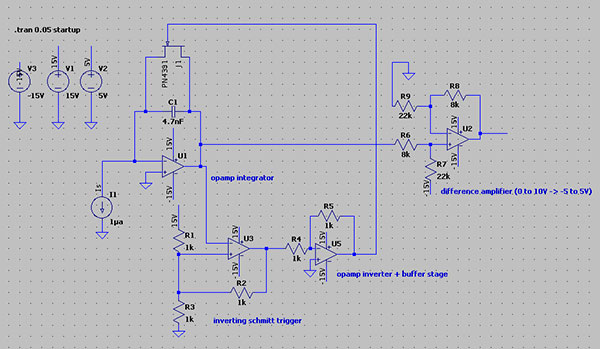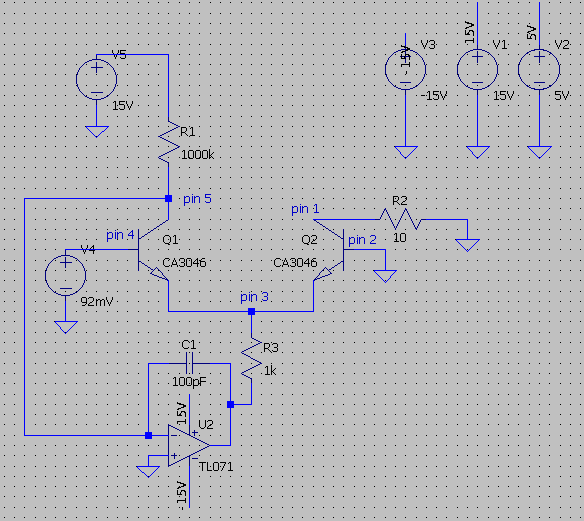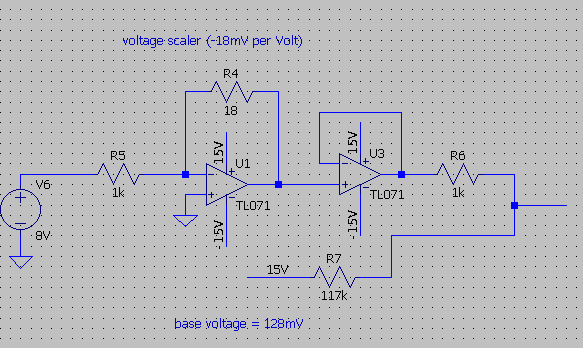# Building an exponential converter

The following table shows the relation between Hertz and wavelength. These are the MIDI C notes per octave, in Hz with their corresponding wavelength in ms

```8.1757989156 hz
122.312205856 ms

16.3515978313 hz
61.156102927 ms

32.7031956626
30.578051464

65.4063913251
15.289025732

130.8127826503
7.644512866

261.6255653006
3.822256433

523.2511306012
1.911128216

1046.5022612024
0.955564108

2093.0045224048
0.477782054
```

The required wavelength a capable VCO should be able to deliver thus lays between 122ms and 0.47ms. Experimenting with a slightly customised sawtooth generator (design below) shows the following relation between the (ideal) current source and the wavelength.

```0.1ua -> 470ms
1ua -> 47ms
10ua -> 4.7ms
100ua -> 0.47ms (470us)
1000ua (1ma)-> 0.047ms
```

First, the slightly customised sawtooth VCO. The inverting Schmitt trigger in the sawtooth reset circuit guarantees a long enough reset pulse and thus a clean reset of the sawtooth waveform. The capacitor is too large, leading to a small distortion in the waveform. Will be fixed in updates.sawtoothvco in PDF

The current source (exponential converter) must deliver a steady current between 0.1ua and 1000ua to the VCO to have to VCO achieve interesting musical abilities (enough octaves, very low frequencies, MIDI controllable). The deployment of an exponential converter in this current source is almost inevitable. Linear control, preferable digital through DAC’s or such, of a current source with such a bandwidth (0.1ua -> 1000ua) is very difficult.

The circuit of the exponential converter:The formula for the exponential part of the converter is iout = iref * exp(-Vb/Vt) where vt = 26mV.

iref runs over R1 => iref = Vref / Rref = 15V / 1000000ohm = 0.000015A = 15uA. To achieve an output current of 0.1ua (the VCO will generate a 470ms waveform) => 0.1 = 15 * exp(-Vb/26) => Vb = 130mV. Some calculations of Vb in the required bandwidth of 0.1ua and 1000ua:

```wavelength: current -> required voltage
470: 0.1ua -> 130mV
235: 0.2ua -> 111mV
118: 0.4ua -> 94mV
59: 0.8ua -> 76mV
30: 1.6ua -> 58mV
15: 3.2ua -> 40mV
8: 5.9ua -> 24mV
4: 11.75ua -> 6mV
2: 24ua -> -12mV
1: 47ua -> -30mV
0.5: 94ua -> -48mV
0.25: 188ua -> -66mV
```

This leads to a relation between linear voltage and exponential current! Required to translate a difference of 1 volt to a musical difference of 1 octave (which is a exponential scale).

The voltage into the exponential converter will thus have a 1V/octave scaling and start at 0 volts (130mV) up to 12 volts (-66mV). The base voltage will be 130mV. The voltage scaling opamp will have to scale the incoming voltage (0-12V) to a -18mV per Volt. This voltage is buffered by an opamp follower and a voltage summer then combines the base voltage and the voltage scaler voltage (note to self: make the 1k in the summer smaller for a more precise summer).The exponential converter takes this voltage (in V4) and transforms it into a current source following calculations above. Temperature drifting and transistor matching have not been explored in full. For now the CA3046 is deployed. Temperature compensation is probably achievable by driving the other transistors in the CA3046 to a certain temp (like in the Moog Prodigy).